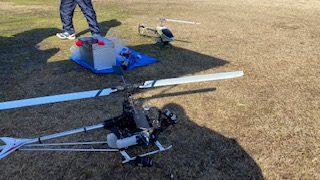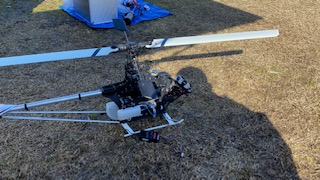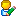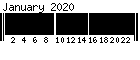## 今日も大盛況です— posted by Nob at 11:00 amComment 

## Mail System Error - Returned Mail

This Message was undeliverable due to the following reason:=E3=81=93=E3=81=AE=E3=83=A1=E3=83=83=E3=82=BB=E3=83=BC=E3=82=B8=E3=81=AF==E3=80=81=E4=BB=A5=E4=B8=8B=E3=81=AE=E7=90=86=E7=94=B1=E3=81=AB=E3=82=88==E3=81=A3=E3=81=A6=E9=85=8D=E9=80=81=E3=81=A7=E3=81=8D=E3=81=BE=E3=81=9B==E3=82=93=E3=81=A7=E3=81=97=E3=81=9F:

Your message is larger than the destination computer is willing toaccept, so it was returned. The error message below indicates thesize of your message and the maximum size allowed by the receivingE-mail system. You may be able to split your message into severalsmaller pieces and have them delivered separately.

Size of this message: 7610438 bytesServer maximum size: 3145728 bytes

The following recipients did not receive this message:

— posted by Nob at 09:58 amComment 

1 2 3 4 5 6 7
8 9 10 11 12 13 14
15 16 17 18 19 20 21
22 23 24 25 26 27 28
29 30 31

Created in 0.1995 sec.
T:Y:ALL:Online: